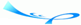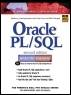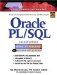# Chapter 5 Conditional Control: IF Statements

 Team-FlyOracle® PL/SQL® Interactive Workbook, Second EditionBy Benjamin Rosenzweig, Elena Silvestrova Table of Contents Appendix A.  Answers to Self-Review Questions

#### Lab 5.1 Self-Review Answers

A1:

Questions

Answers

Comments

1)

C

The statements in an IF construct are not executed sequentially. Rather, one group of statements or another will be selected to execute depending on how a test condition is evaluated.

A2:

Questions

Answers

Comments

2)

A

Only when the condition evaluates to TRUE, the statement of an IF-THEN construct are executed. When the condition evaluates to FALSE or NULL, the control is passed to the first executable statement after the IF-THEN construct. As a result, its statements are not executed at all.

A3:

Questions

Answers

Comments

3)

B

When a condition of the IF-THEN-ELSE construct is evaluated to NULL, the statements specified after keyword ELSE will be executed. In other words, the IF-THEN-ELSE construct behaves as if the condition evaluated to FALSE.

A4:

Questions

Answers

Comments

4)

B

You can specify only two actions in an IF-THEN-ELSE statement. Furthermore, these actions should be mutually exclusive.

A5:

Questions

Answers

Comments

5)

B

The condition of the IF-THEN-ELSE construct can evaluate to TRUE, FALSE, or NULL. When the condition evaluates to TRUE, one group of statements is executed. When the condition evaluates to FALSE or NULL, another group of statement is executed. Hence, the IF-THEN-ELSE construct enables you to specify two and only two mutually exclusive groups of statements.

#### Lab 5.2 Self-Review Answers

A1:

Questions

Answers

Comments

1)

B

An ELSIF construct can have multiple ELSIF clauses, but only one ELSE clause.

A2:

Questions

Answers

Comments

2)

B

A3:

Questions

Answers

Comments

3)

C

The ELSE part is executed when none of the conditions evaluate to TRUE.

A4:

Questions

Answers

Comments

4)

C

As soon as the first condition evaluates to TRUE, statements associated with it are executed. The rest of the ELSIF statement is ignored.

A5:

Questions

Answers

Comments

5)

B

ELSE is an optional part of an ELSIF statement.

#### Lab 5.3 Self-Review Answers

A1:

Questions

Answers

Comments

1)

C

There are no restrictions on how various types of IF statements can be nested one inside another.

A2:

Questions

Answers

Comments

2)

C

A3:

Questions

Answers

Comments

3)

B

You can use different logical operators when writing complex conditions. While there are no restrictions on the number of the logical operators present in a condition, you should be aware of precedence rules. For example,

` x >= 3 OR x <= 5 AND y > 7 `

may produce a result different from

` (x >= 3 OR x <= 5) AND x > 7 `

for the same values of x and y.

A4:

Questions

Answers

Comments

4)

A

There is no need for the conditions of nested IF statements to be mutually exclusive. For example,

` IF v_num > 0 THEN     IF v_num < 0 THEN        …     END IF;  END IF; `

When v_num is greater than 0, the condition of the inner IF statement will evaluate to FALSE. When v_num is less than or equal to 0, the condition of the outer IF statement will evaluate to FALSE. Thus, the inner IF statement will never execute regardless of the value of v_num.

A5:

Questions

Answers

Comments

5)

C

The behavior of an IF statement does not change based on its placement in the block. In other words, if a condition of any IF statement (outer or inner) evaluates to FALSE, the control is always passed to the first executable statement after END IF. It is important to remember that this behavior does not apply to IF-THEN-ELSE and ELSIF.

 Team-FlyTopOracle PL/SQL Interactive Workbook (2nd Edition)
ISBN: 0130473200
EAN: 2147483647
Year: 2002
Pages: 146

Similar book on Amazon

flylib.com © 2008-2017.
If you may any questions please contact us: flylib@qtcs.net